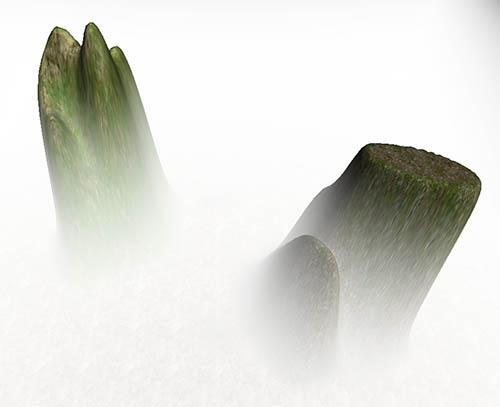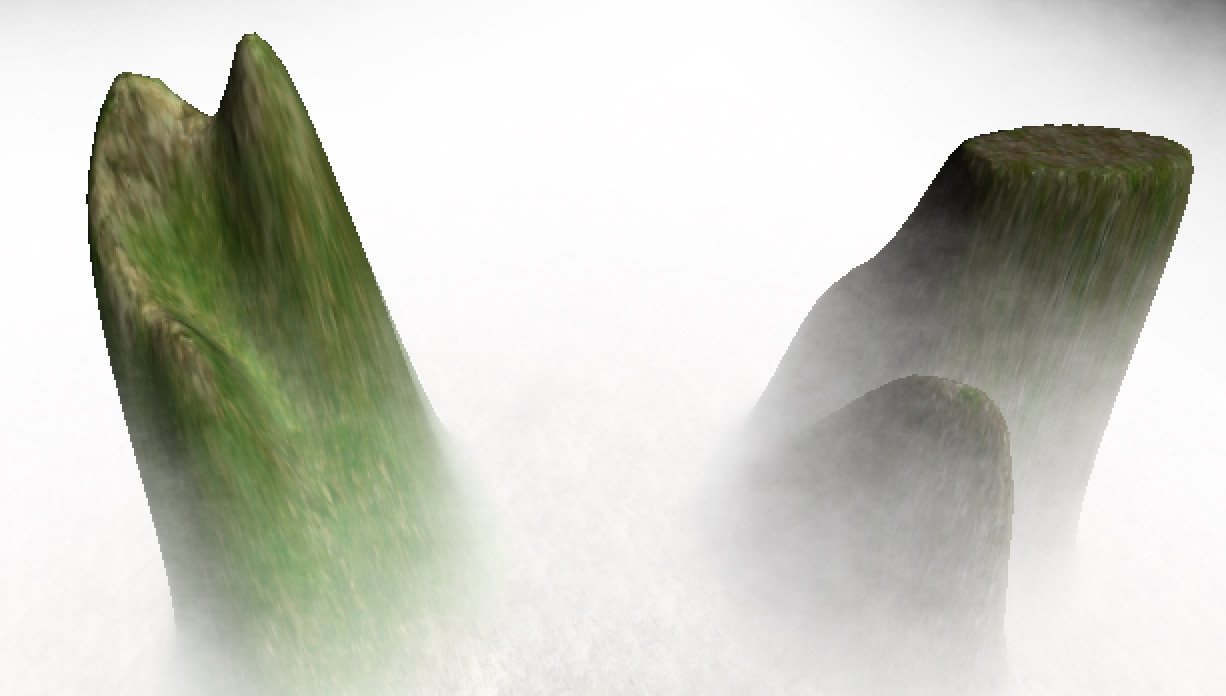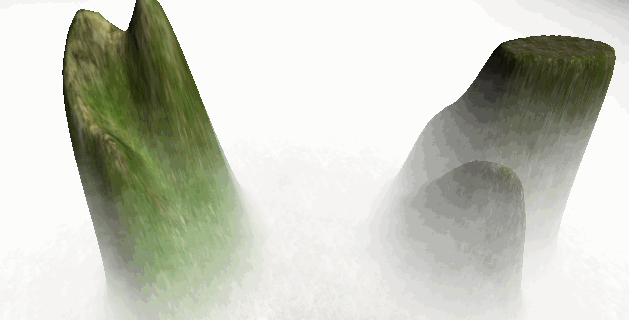# Jim's GameDev Blog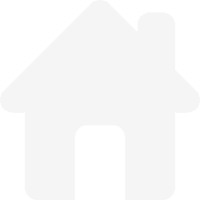# Perlin Noise 及其应用

2016-5-2

Perlin Noise 可以用来表现自然界中无法用简单形状来表达的物体的形态，比如火焰、烟雾、表面纹路等。要生成 Perlin Noise 可以使用工具离线生成，也可以使用代码运行时生成。最简单常用的离线生成工具就是 Photoshop 了，新建画布，然后直接选择云彩滤镜即可。而这里要介绍的是使用代码生成 Perlin Noise，使用代码运行时生成有几点好处。首先就是 Perlin Noise 纹理不会占用空间，如果大量使用到了 Perlin Noise Texture，这就不失为一个减少包大小的好方法，比如说地形的高度图是通过 Perlin Noise 生成的，同时又有很多张地图。还有个好处就是，运行时生成的是可以根据需要产生不同的变化的，而工具离线生成的就做不到。

### 生成一张随机杂点的纹理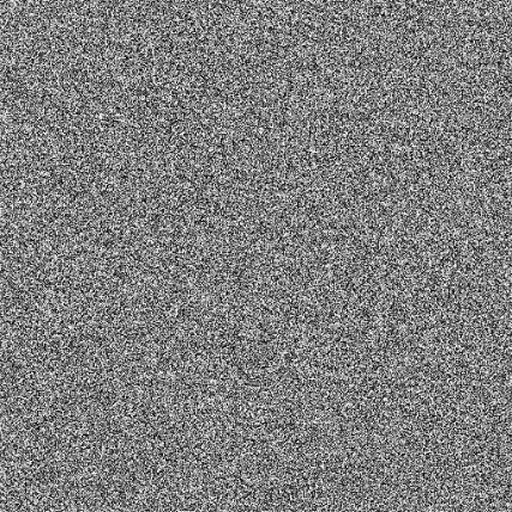``````private float[,] GenerateWhiteNoise(int width, int height)
{
// 这里的随机数种子很重要
// 如果想要每次生成的 Perlin Noise 都一样，只要设置一个相同的值即可
// 这里使用随机种子，所有每次都会生成不同的 Perlin Noise
Random.seed = Random.Range(int.MinValue, int.MaxValue);
float[,] noise = new float[width, height];

for (int i = 0; i < width; i++)
{
for (int j = 0; j < height; j++)
{
noise[i, j] = Random.value;
}
}

return noise;
}
``````

### 使用不同的采样率对随机杂点纹理进行采样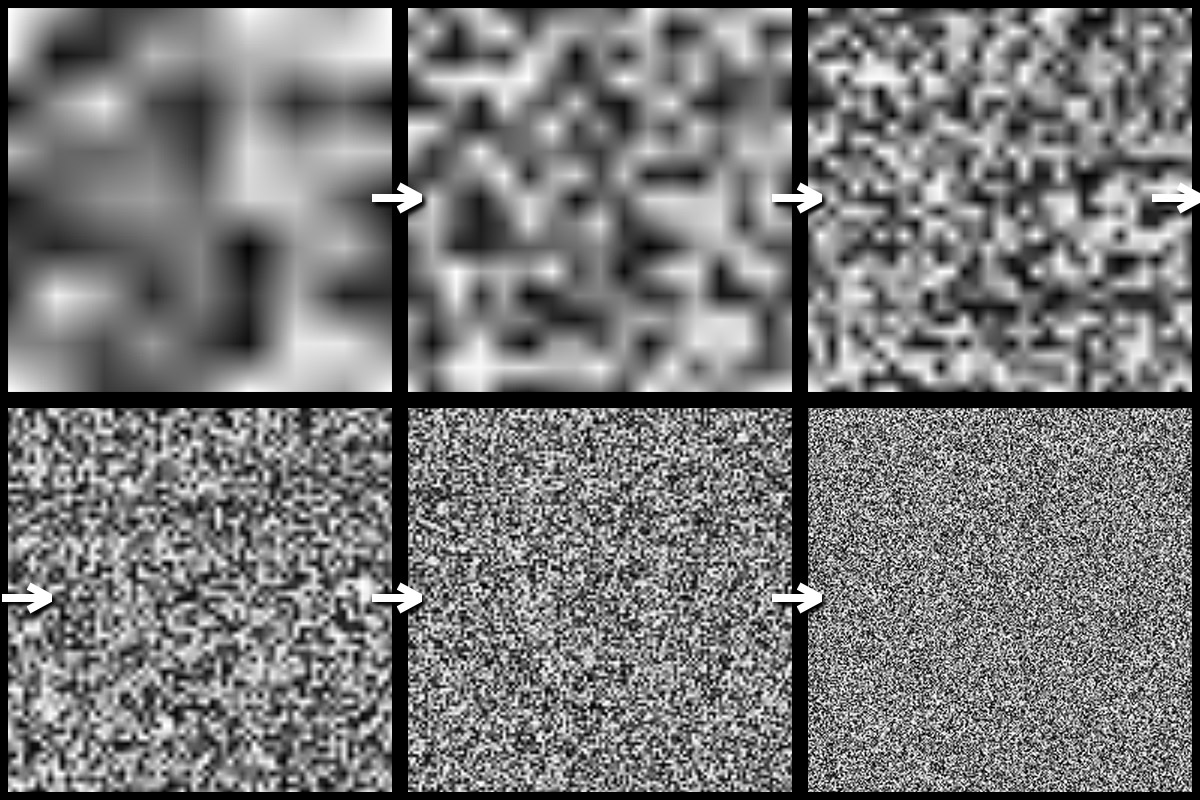``````// 生成指定采样级别的 Noise
private float[,] GenerateSmoothNoise(float[,] whiteNoise, int octave, int width, int height)
{
float[,] smoothNoise = new float[width, height];

// 采样步长
int samplePeriod = (int)Mathf.Pow(2, octave);
// 采样频率
float sampleFrequency = 1.0f / samplePeriod;

for (int i = 0; i < width; i++)
{
// 最左点位置
int sampler_l = (i / samplePeriod) * samplePeriod;
// 最右点位置
int sampler_r = (sampler_l + samplePeriod) % width;
// 根据实际点与最左最右的距离，计算水平混合系数
float horizontal_blend = (i - sampler_l) * sampleFrequency;

for (int j = 0; j < height; j++)
{
// 最上点位置
int sampler_t = (j / samplePeriod) * samplePeriod;
// 最下点位置
int sampler_d = (sampler_t + samplePeriod) % height;
// 根据实际点与最上最下的距离，计算垂直混合系数
float vertical_blend = (j - sampler_t) * sampleFrequency;

// 左上和右上根据水平混合系数进行插值
float top = Interpolate(whiteNoise[sampler_l, sampler_t], whiteNoise[sampler_r, sampler_t], horizontal_blend);
// 左下和右下根据水平混合系数进行插值
float bottom = Interpolate(whiteNoise[sampler_l, sampler_d], whiteNoise[sampler_r, sampler_d], horizontal_blend);
// 最总数值为 top down 根据垂直混合系数进行插值
smoothNoise[i, j] = Interpolate(top, bottom, vertical_blend);
}
}

return smoothNoise;
}

// 在两个数值间进行插值
private float Interpolate(float x0, float x1, float alpha)
{
return x0 * (1 - alpha) + alpha * x1;
}
``````

### 合成最终的 Perlin Noise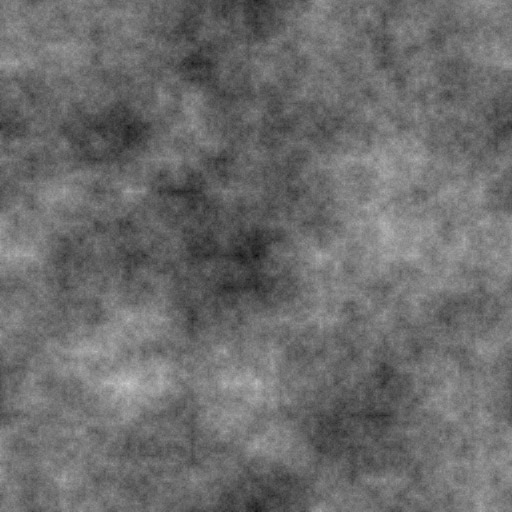``````public float[,] GeneratePerlinNoise(float[,] whiteNoise, int octaveCount, int width, int height)
{
// 不同采样级别的 Noise
List<float[,]> smoothNoise = new List<float[,]>();

// 不同采样级别的 Noise 的叠加系数
float persistance = 0.8f;

// 生成不同采样级别的 Noise
for (int i = 0; i < octaveCount; i++)
{
}

// 最终生成的 Perlin Noise
float[,] perlinNoise = new float[width, height];

// 不同采样级别的 Noise 的叠加比重
float amplitude = 1.0f;
// 所有采样级别的 Noise 的叠加总比重
float totalAmplitude = 0.0f;

// 开始混合所有采样级别的 Noise
for (int octave = octaveCount - 1; octave >= 0; octave--)
{
amplitude *= persistance;
totalAmplitude += amplitude;

for (int i = 0; i < width; i++)
{
for (int j = 0; j < height; j++)
{
perlinNoise[i,j] += smoothNoise[octave][i,j] * amplitude;
}
}
}

// 归一化最终的 Perlin Noise
for (int i = 0; i < width; i++)
{
for (int j = 0; j < height; j++)
{
perlinNoise[i,j] /= totalAmplitude;
}
}

return perlinNoise;
}
``````

### 应用：残蚀效果

_ _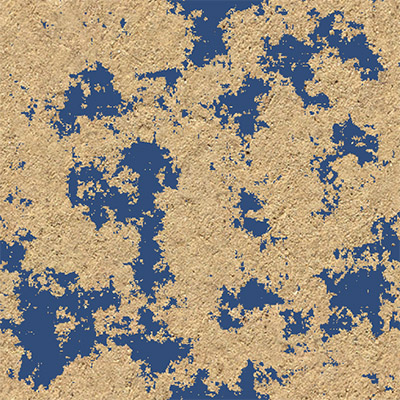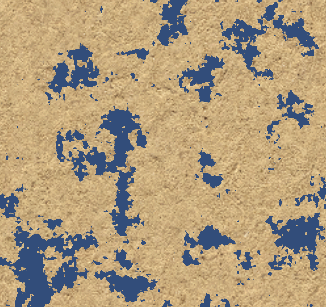### 应用：动态体积雾